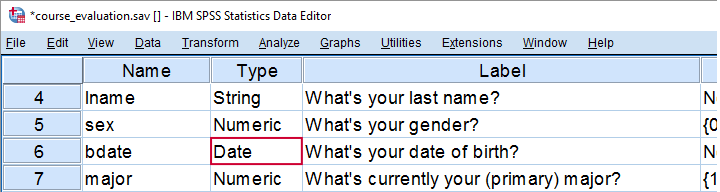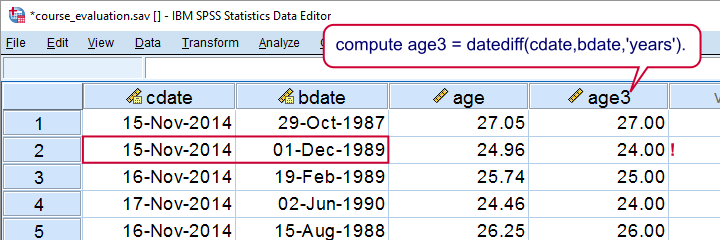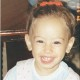# SPSS tutorials

BASICS DATA ANALYSIS T-TEST ANOVA CHI-SQUARE TEST

# How to Compute Age in SPSS?

A course was evaluated by 183 students. The data are in course_evaluation.sav, part of which is shown below. The teacher wants to know the average age of his students but we only have their date of birth.## 1. Ensure Date of Birth is a Date Variable

The first thing we'll do is check if date of birth is a real date variable. We readily see in variable view that this is the case here. Sometimes dates end up in SPSS as string variables and if so, we first need to convert them to date variables. Some examples for doing so are in Convert String to Date Variable.

## 2. Choose a Comparison Date

Since (average) age is literally changing every second, we need to answer “age at which point in time?” The most obvious option is age at the moment the data were collected. Such a completion date may be present in your data. If it isn't, we'll make an educated guess.

## 3. Compute Age with Known Completion Date

Our data hold a variable cdate which contains the completion dates for the questionnaire. We'll now easily compute age with the syntax below and we'll inspect its histogram to make sure the result has a plausible distribution.

*Compute age if completion date known.

compute age = datediff(cdate,bdate,'days') / 365.25.

*Inspect if result has plausible distribution.

frequencies age
/format notable
/histogram.

*All ages between 19 and 27 years. Looks perfect.

## ResultSo we basically computed the number of days between date of birth and completion and divided that by 365.25, the average number of days in a year. You may wonder why we don't just use DATEDIFF(cdate,bdate,'years'). We'll get to that in a minute.

## 4. Compute Age with Unknown Completion Date

If we don't have a completion date in our data, we'll try and make a good guess. Let's say we guess January 1, 2015. We can convert this into an SPSS date value by using date.dmy(1,1,2015) and thus create our guessed completion date as a new variable in our dataset. Alternatively, we may insert this function directly into our age computation formula as shown below.

*Compute age if completion date must be guessed.

compute age2 = datediff(date.dmy(1,1,2015),bdate,'days') / 365.25.
execute.

## Days or Years?

So why did we extract days and divide those by 365.25, the average number of days in a year? The simple reason is that SPSS truncates the outcome of DATEDIFF. This means that someone who is 20 years and 364 days old will be assigned an age of 20.00 years, which is almost an entire year off.

*Compute age - wrong way.

compute age3 = datediff(cdate,bdate,'years').
execute.

## ResultThis probably convinces you that extracting years directly is not a good idea: on average, we'll underestimate age by half a year by doing so.For the sake of simplicity, we'll assume that birthdays are uniformly distributed over the year, which I believe roughly holds.

## Final Notes

If you don't want to see any decimal places, your best option is probably running formats age (f3). which will display all ages as integers. Alternatively, if you want ages to be integers, you could run compute age = rnd(age). but this obviously introduces some error -bad but not quite as bad as the aforementioned bias.

# Let me know what you think!

*Required field. Your comment will show up after approval from a moderator.

# This tutorial has 38 comments

•### By William Peck on November 2nd, 2018

I always truncate the partial year, that's the common way of presenting age, since you're not 18 until you're 18, you're 17 ... :-)

Good explanation and I like that the datasets are like 95% match to the web page. I'm on v25.

•### By Ruben Geert van den Berg on September 5th, 2017

Hi Heba!

The first thing that comes to mind is that dates with 2-digit years can look different from what they are. I mean that "01-02-03" probably means 1 February 2003 but it could be 1 February 1903, 1803 or basically anything. I've encountered this problem with real life data (imported from Excel I think). You can check if this is the case by running something like

`FORMATS BIRTHDAY (DATE11).`

This does not change anything about your data. It just displays it differently. If that's not the problem, share a handful of your data with me and I'll look into them.

•### By Heba on September 5th, 2017

I am trying to convert birthdate into age using exactly this. It has worked, however certain age variables keep coming out incorrect and in negative. I checked my input and it's all correct, what could be the problem or how to do I correct that?

•### By Jon Peck on April 6th, 2017

Datediff is designed to do exact calculations in the same way that, say, the Motor Vehicle Department would: you are 20 until your 21st birthday. Dividing by 365.25 is fine for most statistical purposes but, of course, does not adjust exactly for leap years. Age in days would work for statistics, too.

•good